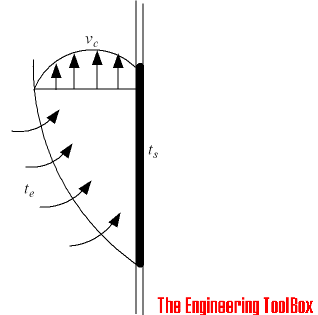Engineering ToolBox - Resources, Tools and Basic Information for Engineering and Design of Technical Applications!

# Convective Heat Transmission - Air Velocity and Air Flow Volume

## Hot or cold vertical surfaces generates vertical air flows - calculate air velocity and volume flow.

A hot or cold vertical surface will generate a vertical upwards or downwards air flow as indicated in the figure below.The air velocity in the center of a convective air flow in parallel to the hot or cold surface - at a vertical distance - can be estimated as:

### Air Velocity

vc = 0.65 [g l dt / (273 + te)]1/2                                              (1)

where

vc = velocity in center of airflow (m/s)

g = acceleration of gravity (9.81 m/s2)

l = vertical distance from bottom (or top) of the surface (m)

dt = te - ts= temperature difference between surface and room environment

ts = surface temperature (oC)

te = surrounding environment - ambient - temperature (oC)

For a cold surface where the air will flow downwards - the value will be negative.

### Air Volume

Convective air flow volume at a vertical distance generated by the hot or cold surface can be calculated as

Q = 0.019 [g (te - ts) / (273 + te)]0.4 l1.2                                            (2)

where

Q = air flow volume (m3/s per meter surface width)

### Example - Convective Air Flow from a Cold Window

The air velocity at the lower level of a cold (5oC) window with height 2 m in a room with temperature 20oC can be calculated as

vc = 0.65 [(9.81 m/s2) (2 m) ((20 oC) - (5 oC)) / (273 + (20 oC))]1/2

= 0.65 m/s

The air flow volume (for a window with width 1m) can be calculated as

Q = 0.019 [(9.81 m/s2) ((20 oC) - (5 oC) / (273 + (20 oC))]0.4 (2 m)1.2

= 0.033 m3/s

= 119 m3/h

### Example - A Heating Element (or Radiator) and Convective Air Flow

The air flow velocity at the top of a hot (80oC) radiator with height 1 m in a room with temperature 20oC can be calculated as

vc = 0.65 [(9.81 m/s2) (1 m) ((80 oC) - (20 oC) / ((273 K) + (20 oC))]1/2

= 0.92 m/s

The air flow volume (for radiator with width 1 m) can be calculated as

Q = 0.019 [(9.81 m/s2) ((80 oC) - (20 oC) / ((273 K) + (20 oC))]0.4 (1 m)1.2

= 0.025 m3/s

= 90.4 m3/h

## Related Topics

• Heating - Heating systems - capacity and design of boilers, pipelines, heat exchangers, expansion systems and more.

## Engineering ToolBox - SketchUp Extension - Online 3D modeling!

Add standard and customized parametric components - like flange beams, lumbers, piping, stairs and more - to your Sketchup model with the Engineering ToolBox - SketchUp Extension - enabled for use with the amazing, fun and free SketchUp Make and SketchUp Pro .Add the Engineering ToolBox extension to your SketchUp from the SketchUp Pro Sketchup Extension Warehouse!

Translate

## Privacy

We don't collect information from our users. Only emails and answers are saved in our archive. Cookies are only used in the browser to improve user experience.

Some of our calculators and applications let you save application data to your local computer. These applications will - due to browser restrictions - send data between your browser and our server. We don't save this data.

## Citation

• Engineering ToolBox, (2005). Convective Heat Transmission - Air Velocity and Air Flow Volume. [online] Available at: https://www.engineeringtoolbox.com/convective-air-flow-d_1006.html [Accessed Day Mo. Year].

Modify access date.

. .

#### Scientific Online Calculator6 24